# Resources tagged with: Vector Notation and Geometry

Filter by: Content type:
Age range:
Challenge level:

There are 20 NRICH Mathematical resources connected to Vector Notation and Geometry, you may find related items under Vectors and Matrices.

Broad Topics > Vectors and Matrices > Vector Notation and Geometry### Vector Racer

##### Age 11 to 16Challenge Level

The classic vector racing game.### Vector Journeys

##### Age 14 to 18Challenge Level

Charlie likes to go for walks around a square park, while Alison likes to cut across diagonally. Can you find relationships between the vectors they walk along?### Vector Walk

##### Age 14 to 18Challenge Level

Starting with two basic vector steps, which destinations can you reach on a vector walk?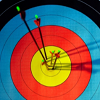### Spotting the Loophole

##### Age 14 to 16Challenge Level

A visualisation problem in which you search for vectors which sum to zero from a jumble of arrows. Will your eyes be quicker than algebra?### Areas of Parallelograms

##### Age 14 to 16Challenge Level

Can you find the area of a parallelogram defined by two vectors?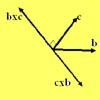### Multiplication of Vectors

##### Age 16 to 18

An account of multiplication of vectors, both scalar products and vector products.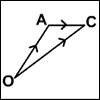### An Introduction to Vectors

##### Age 14 to 18

The article provides a summary of the elementary ideas about vectors usually met in school mathematics, describes what vectors are and how to add, subtract and multiply them by scalars and indicates. . . .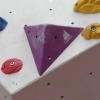### Tetra Perp

##### Age 16 to 18Challenge Level

Show that the edges AD and BC of a tetrahedron ABCD are mutually perpendicular when: AB²+CD² = AC²+BD².### Flexi Quads

##### Age 16 to 18Challenge Level

A quadrilateral changes shape with the edge lengths constant. Show the scalar product of the diagonals is constant. If the diagonals are perpendicular in one position are they always perpendicular?### Vector Gem Collector

##### Age 14 to 18Challenge Level

Use vectors to collect as many gems as you can and bring them safely home!### From Point to Point

##### Age 14 to 16 ShortChallenge Level

Can you combine vectors to get from one point to another?### Matrix Meaning

##### Age 16 to 18Challenge Level

Explore the meaning behind the algebra and geometry of matrices with these 10 individual problems.### Polygon Walk

##### Age 16 to 18Challenge Level

Go on a vector walk and determine which points on the walk are closest to the origin.### Coordinated Crystals

##### Age 16 to 18Challenge Level

Explore the lattice and vector structure of this crystal.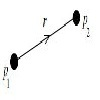### Hold Still Please

##### Age 16 to 18Challenge Level

Can you arrange a set of charged particles so that none of them start to move when released from rest?### Quaternions and Rotations

##### Age 16 to 18Challenge Level

Find out how the quaternion function G(v) = qvq^-1 gives a simple algebraic method for working with rotations in 3-space.### Spiroflowers

##### Age 16 to 18Challenge Level

Analyse these repeating patterns. Decide on the conditions for a periodic pattern to occur and when the pattern extends to infinity.### Triangle in a Triangle

##### Age 14 to 16Challenge Level

Can you work out the fraction of the original triangle that is covered by the inner triangle?### Napoleon's Theorem

##### Age 14 to 18Challenge Level

Triangle ABC has equilateral triangles drawn on its edges. Points P, Q and R are the centres of the equilateral triangles. What can you prove about the triangle PQR?### A Knight's Journey

##### Age 14 to 18

This article looks at knight's moves on a chess board and introduces you to the idea of vectors and vector addition.